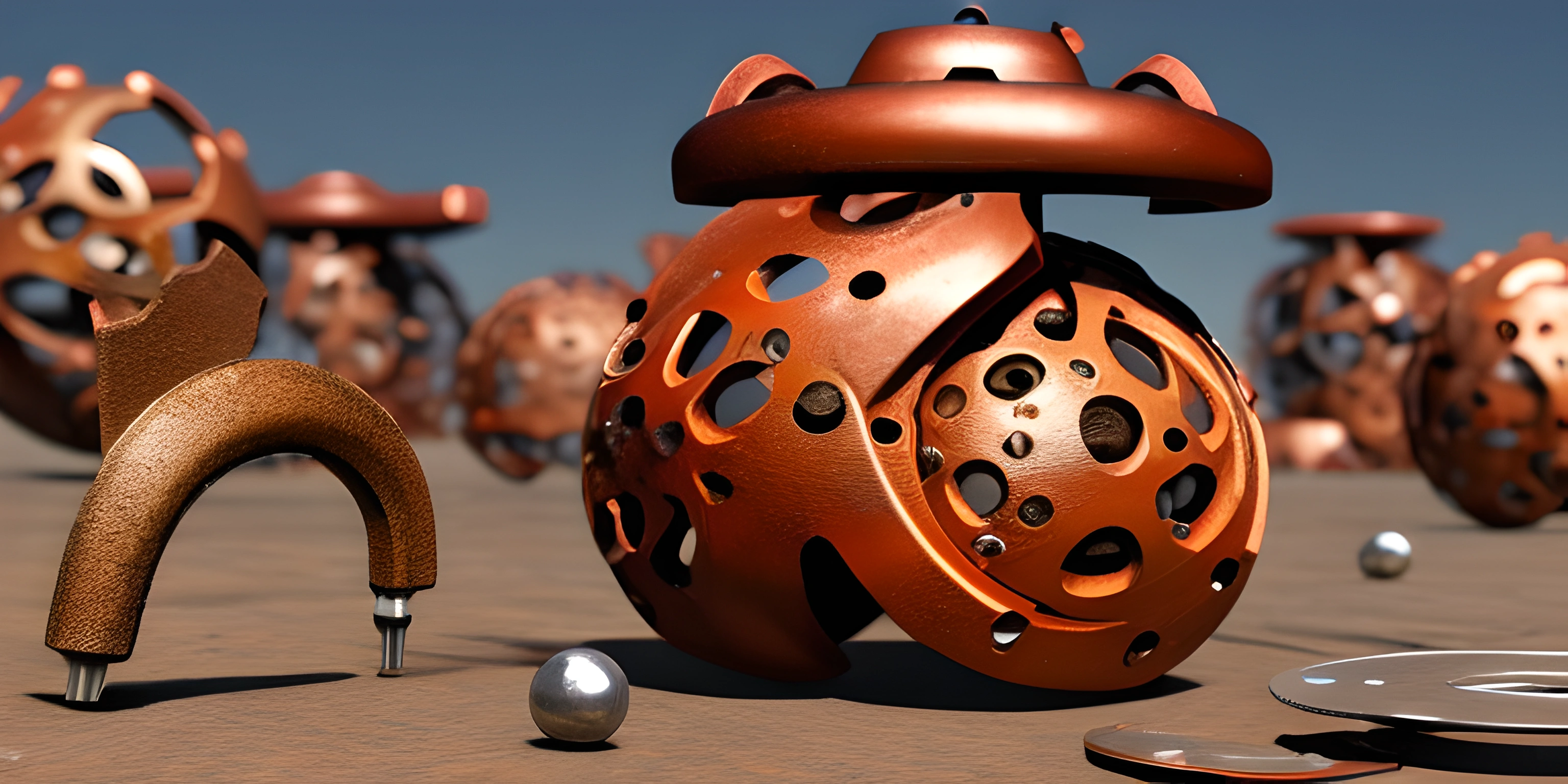# Understanding Rust VectorsNote: this page has been created with the use of AI. Please take caution, and note that the content of this page does not necessarily reflect the opinion of Cratecode.

Vectors are a powerful and essential data structure in the Rust programming language. A vector is a growable array that can hold elements of the same type. They are the go-to choice for dynamic, resizable collections because of their versatility and efficiency.

## Creating Vectors

Creating a vector in Rust is simple. You can either use the `vec!` macro or the `Vec::new()` method:

``````let mut v1 = vec![1, 2, 3];
let mut v2: Vec<i32> = Vec::new();``````

In the example above, `v1` is created using the `vec!` macro with three elements, while `v2` is an empty vector of type `i32`, created using `Vec::new()`.

To add elements to a vector, you can use the `push` method. This method appends an element to the end of the vector:

``````let mut v = vec![1, 2, 3];
v.push(4);
v.push(5);``````

After the code above runs, `v` will contain the elements `[1, 2, 3, 4, 5]`.

## Accessing Elements

You can access elements in a vector using either indexing or the `get` method:

``````let v = vec![1, 2, 3];

// Indexing
let value1 = v; // 2

// Using the 'get' method
let value2 = v.get(1); // Some(2)``````

Indexing returns a reference to the value at the specific index, while the `get` method returns an `Option`, which will be `Some(value)` if the index is valid or `None` if the index is out of bounds.

## Iterating Through Elements

You can efficiently iterate through the elements of a vector using a `for` loop:

``````let v = vec![1, 2, 3];

for value in &v {
println!("Value: {}", value);
}``````

This code will print out:

``````Value: 1
Value: 2
Value: 3``````

You can also iterate through mutable references if you need to modify the elements:

``````let mut v = vec![1, 2, 3];

for value in &mut v {
*value *= 2;
}

println!("{:?}", v); // [2, 4, 6]``````

This code multiplies each element in the vector by 2, resulting in a vector containing `[2, 4, 6]`.

## Removing Elements

You can remove elements from a vector using the `pop` method, which removes and returns the last element as an `Option`:

``````let mut v = vec![1, 2, 3];
let last = v.pop(); // Some(3)``````

In the example above, the `pop` method removes the last element (3) from the vector `v` and returns it as `Some(3)`.

## Conclusion

Vectors are versatile and powerful data structures in Rust, allowing for dynamic, resizable collections of elements. From creating and manipulating vectors to accessing and iterating through their elements, understanding how to use vectors effectively is crucial in Rust programming. Happy coding!

## FAQ

### What is a Rust vector and why should I use it?

A Rust vector is a dynamic, growable array that can store elements of the same type. It is part of Rust's standard library, and it's a highly efficient and flexible data structure. You should use Rust vectors when you need to store a collection of elements that can change in size or when you need fast access to elements by index.

### How do I create a new vector in Rust?

Creating a new vector in Rust is easy. You can use the `vec!` macro or the `Vec::new` method. Here are two examples:

``````// Using the vec! macro
let my_vector = vec![1, 2, 3];
// Using the Vec::new method
let mut another_vector = Vec::new();
another_vector.push(1);
another_vector.push(2);
another_vector.push(3);``````

### How can I access and modify elements in a Rust vector?

You can access elements in a Rust vector using indexing or the `get` method. To modify elements, you can use indexing with a mutable reference or the `get_mut` method. Here are examples:

``````let mut my_vector = vec![1, 2, 3];
// Accessing elements with indexing
let first_element = my_vector;
// Accessing elements with the get method
let second_element = my_vector.get(1).unwrap();
// Modifying elements with indexing
my_vector = 4;
// Modifying elements with get_mut
if let Some(elem) = my_vector.get_mut(1) {
*elem = 5;
}``````

### How can I iterate through the elements of a Rust vector?

You can iterate through the elements of a Rust vector using a `for` loop and different types of references. Here are some examples:

``````let my_vector = vec![1, 2, 3];
// Iterating with an immutable reference
for elem in &my_vector {
println!("{}", elem);
}
// Iterating with a mutable reference
let mut another_vector = vec![4, 5, 6];
for elem in &mut another_vector {
*elem += 1;
println!("{}", elem);
}``````

### How can I remove or add elements to a Rust vector?

To add elements to a Rust vector, you can use the `push` or `insert` methods. To remove elements, you can use the `pop`, `remove`, or `truncate` methods. Here are examples:

``````let mut my_vector = vec![1, 2, 3];